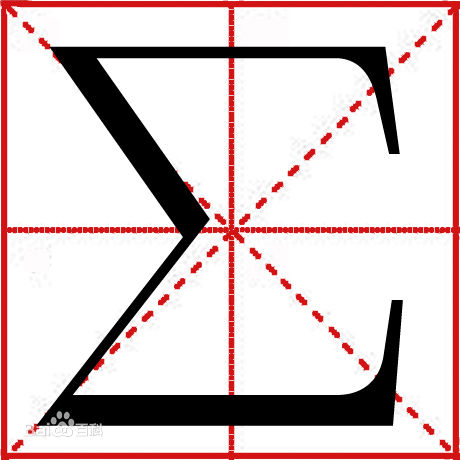# ∑符号解释∑ (求和符号）

∑ 是加起来的意思. 例如

5
∑ ( i ) = (1) + (2) + (3) + (4) + (5) = 1 + 2 + 3 + 4 + 5 = 15
i=1

4
∑ ( i x i ) = (2x2) + (3x3) + (4x4) = 4 + 9 + 16 = 29
i = 2